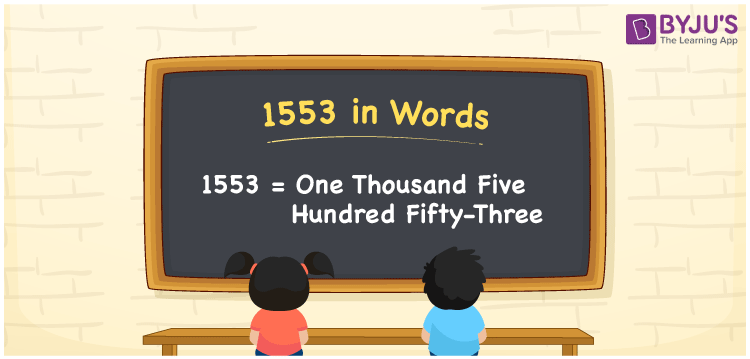# 1553 in Words

1553 in words is one thousand five hundred fifty-three. Using the ones, tens and hundreds places of a number, we can easily convert the number 1553 into words. In Mathematics, 1553 is a natural number and it occurs between 1552 and 1554. In this article, let us discuss how to spell 1553 in words using the place value system in detail.

 1553 in Words: One Thousand Five Hundred Fifty-three. One Thousand Five Hundred Fifty-three in Numerical Form: 1553.

## 1553 in English Words## How to Write 1553 in Words?

Following is the place value table for the number 1553.

 Thousands Hundreds Tens Ones 1 5 5 3

The expanded form of 1553 is as follows:

= 1 × Thousand + 5 × Hundred + 5 × Ten + 3 × One

= 1 × 1000 + 5 × 100 + 5 × 10 + 3 × 1

= 1000 + 500 + 50 + 3

= 1553

= One thousand five hundred fifty-three

Hence, 1553 in words is one thousand five hundred fifty-three.

1553 in words – One thousand five hundred fifty-three

Is 1553 an odd number? – Yes

Is 1553 an even number? – No

Is 1553 a perfect square number? – No

Is 1553 a perfect cube number? – No

Is 1553 a prime number? – Yes

Is 1553 a composite number? – No

## Frequently Asked Questions on 1553 in Words

Q1

### How to write 1553 in words?

1553 in words is one thousand five hundred fifty-three.

Q2

### Simplify 1500 + 53, and express it in words.

Simplifying 1500 + 53, we get 1553. Hence, 1553 in words is one thousand five hundred fifty-three.

Q3

### Is 1553 a prime number?

Yes, 1553 is a prime number.